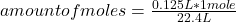## A 125 ml sample of gas is at STP how many moles of gas are in the sample

Question

A 125 ml sample of gas is at STP how many moles of gas are in the sample

in progress 0
6 months 2021-08-04T09:24:27+00:00 2 Answers 9 views 0

5.58*10⁻³ moles of gas are in the sample.

Explanation:

The STP conditions refer to the standard temperature and pressure. Pressure values at 1 atmosphere and temperature at 0 ° C are used and are reference values for gases. And in these conditions 1 mole of any gas occupies an approximate volume of 22.4 liters.

Being 1000 mL equivalent to 1 L, then 125 mL is equal to 0.125 L. Then you can apply the following rule of three: if by STP conditions 22.4 L are occupied by 1 mole, 0.125 L are occupied by how many moles?aomunt of moles= 5.58*10⁻³ moles

5.58*10⁻³ moles of gas are in the sample.

5.58×10⁻³ moles of gas

Explanation:

There is a rule that says, that 1 mol of any gas, at STP is contained at 22.4L of volume.

We can apply a conversion factor

0.125 L . 1 mol / 22.4 L = 5.58×10⁻³ moles

Notice we converted volume from mL to L

We can also aply the Ideal Gases Law.

At STP we have 1 atm of pressure and 273.15K of T°

We replace data: 0.125 L . 1 atm = n . 0.082 . 273.15K

(0.125 L . 1 atm) / (0.082 . 273.15 K) = n

n = 5.58×10⁻³ moles